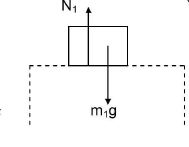# Free Body Diagram – What is FBD and How to draw an FBD?

This post will tell you what a Free Body Diagram or FBD is. Also, you will get a step-by-step guide on How to draw an FBD while solving a problem in physics (mechanics).

In physics, most problems are solved much more easily when a free body diagram is used. This uses geometry and vectors to visually represent the problem, and trigonometry is also used in determining horizontal and vertical components of forces and objects.

## What is a Free Body Diagram (FBD)? | Purpose of Free body diagram

In a free body diagram (FBD), all the forces acting on a body are shown with their magnitudes and direction. The purpose of the FBD is to ensure that we consider all the forces acting on the body properly without missing out on any. If there is more than one body in the problem, each body requires an FBD. In general, any body having mass needs one FBD.

Purpose of FBD: Free body diagrams are very helpful in visually identifying which components are unknown, where the moments are applied, and help analyze a problem, whether static or dynamic.

## How to draw a Free Body Diagram or FBD?

We can draw an FBD by following the steps listed below:

1. To draw a free body diagram, do not worry about drawing it to scale
2. Draw the body (that we want to study) as a point mass or a small block etc.
3. Show the immediate surroundings (i.e. the objects/bodies in contact with the subject body) preferably in a dotted line.
4. List out the various forces experienced by the body, disregarding the forces exerted by the body because they will appear as forces experienced by some other body in the system.
5. Now draw all the forces acting on the body as arrows with directions clearly marked. (because the motion of a body is solely determined by forces acting on it)
6. Ignore forces exerted by this body on others,
7. Show, by means of arrows, alongside the body, the parameters of motion of the body (velocity/acceleration) with direction.

## Sample Free Body Diagram or FBDfigure 1: FBD of a block resting on another block ( forces shown are gravity and normal reaction)